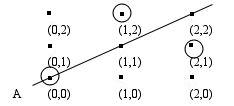## Supplement: Finite Fields as Models for Euclidean Plane Geometry

### Finite Fields

A field is a set $$F$$ equipped with two operations +, · such that

1. the pair $$\langle F,+\rangle$$ is a group, i.e.,
 + is closed: $$\forall x,y\exists z(x + y = z)$$ + is associative: $$\forall x,y,z(x + (y + z) = (x + y) + z)$$ there is a neutral element: $$\forall x(x + 0 = x)$$ every element has an inverse: $$\forall x\exists y(x + y = y + x = 0)$$
2. likewise $$\langle F,\cdot \rangle$$ is a group, the neutral element being 1
3. the following distributivity laws hold: \begin{align*} \forall x,y,z(x \cdot (y + z) &= (x \cdot y) + (y \cdot z))\\ \forall x,y,z((x + y) \cdot z) &= (x \cdot z) + (y \cdot z)) \end{align*}

It is straightforward to see that the real numbers $$\Re$$ with the usual addition and multiplication is a field. In this case the set $$F$$ is infinite, but $$F$$ can be finite as well. Then we have a finite field or a Galois field. There is however one very important distinction between a field such as $$\Re$$ and a Galois field. In the latter, given the multiplicative neutral element 1, there is a prime number $$p$$ such that $$p \cdot 1 = 0$$. $$p$$ is called the characteristic of the field. It can be shown that if $$p$$ is the characteristic of a field, then it must have $$p^{n}$$ elements, for some natural number $$n$$. In addition Galois fields are the only finite fields.

Example: the Galois field with characteristic 3 and number of elements 3, $$GF(3)$$ for short.

The tables for addition and multiplication tell the whole story:

 + 0 1 2 0 0 1 2 1 1 2 0 2 2 0 1

 · 0 1 2 0 0 0 0 1 0 1 2 2 0 2 1

In the first case, $$1 + 1 + 1 = 0$$.

It is easy to see that the above tables correspond to addition and multiplication modulo $$p$$. In other words, in the field $$a = b$$ if and only if $$a \equiv b(\mathrm{mod}\, p)$$.

### Euclidean Axioms in a Finite Field

To see how a finite field can be a model, I will take the incidence relation. The axioms for the incidence relation are:

1. Through two points exactly one straight line can be drawn.
2. There are at least two points on every straight line.
3. There are at least three points that are not on the same straight line.

In case we take $$GF(3)$$ as a possible model, then to a point $$p$$ corresponds to a couple $$(x,y)$$, such that $$0\le x,y\le 2$$. A line corresponds to a triple $$(a,b,c)$$, such that $$0\le a,b,c\le 2$$ and at most one of $$a = 0$$ or $$b = 0$$, or, equivalently, at most one of $$a\equiv 0$$ or $$b\equiv 0 (\mathrm{mod}\, 3)$$. The incidence relation is translated as follows:

a point $$p$$ lies on a line $$A$$ iff if $$(x,y)$$ corresponds to $$p$$ and $$(a,b,c)$$ corresponds to $$A$$, then the equation $$ax+by+c = 0$$ is satisfied, or, equivalently, $$ax+by+c\equiv 0 (\mathrm{mod}\, 3)$$ is satisfied.

Some facts are now straightforward to check:

1. There are exactly $$p^{2}$$ points.
2. There are exactly $$p(p+1)$$ straight lines.
3. On every straight line there are exactly $$p$$ points.
4. Through every point pass exactly $$p+1$$ straight lines.
5. The axioms (a), (b) and (c) are true in this model.

A graphical representation could look like this:Figure 5.

The line indicated by $$A$$ corresponds to $$x = y$$. This seems to correspond nicely to the classical Euclidean case. However something strange happens with the linear equation $$x + y = 0$$. In the drawing the 3 points that are on this line have been circled. As must be clear these lines are “bad” and should be "ignored" in the model.

In order to satisfy the remaining axioms further restrictions are required on the size of the domain. These will just be mentioned without details:

1. $$p$$ must have the specific form $$4n+3$$ (and not $$4n+1)$$.
2. $$p$$ must in addition have the specific form $$8mq_{1}q_{2}\ldots q_{k} - 1$$, where $$q_{i}$$ is the $$i$$-th odd prime (so $$q_{1} = 3)$$ and $$m$$ a positive integer.

The second condition that is needed to guarantee the existence of the Euclidean “kernel” is a non-trivial statement. It actually requires some essential parts of number theory to prove that there are prime numbers of that form. There are, classically speaking, an infinite number of them. By choosing $$p$$ large enough, one can make the “kernel” as large as one desires to have the Euclidean approximation as close as one wants.

Note: As Ernst Welti points out, it would be a rather annoying situation for a finitist if the proof that shows that there are an infinite number of primes of the right form were not finitistically acceptable. Although the original proof of Dirichlet was in fact unacceptable, fortunately there does now exist a finitistically acceptable proof of the theorem.